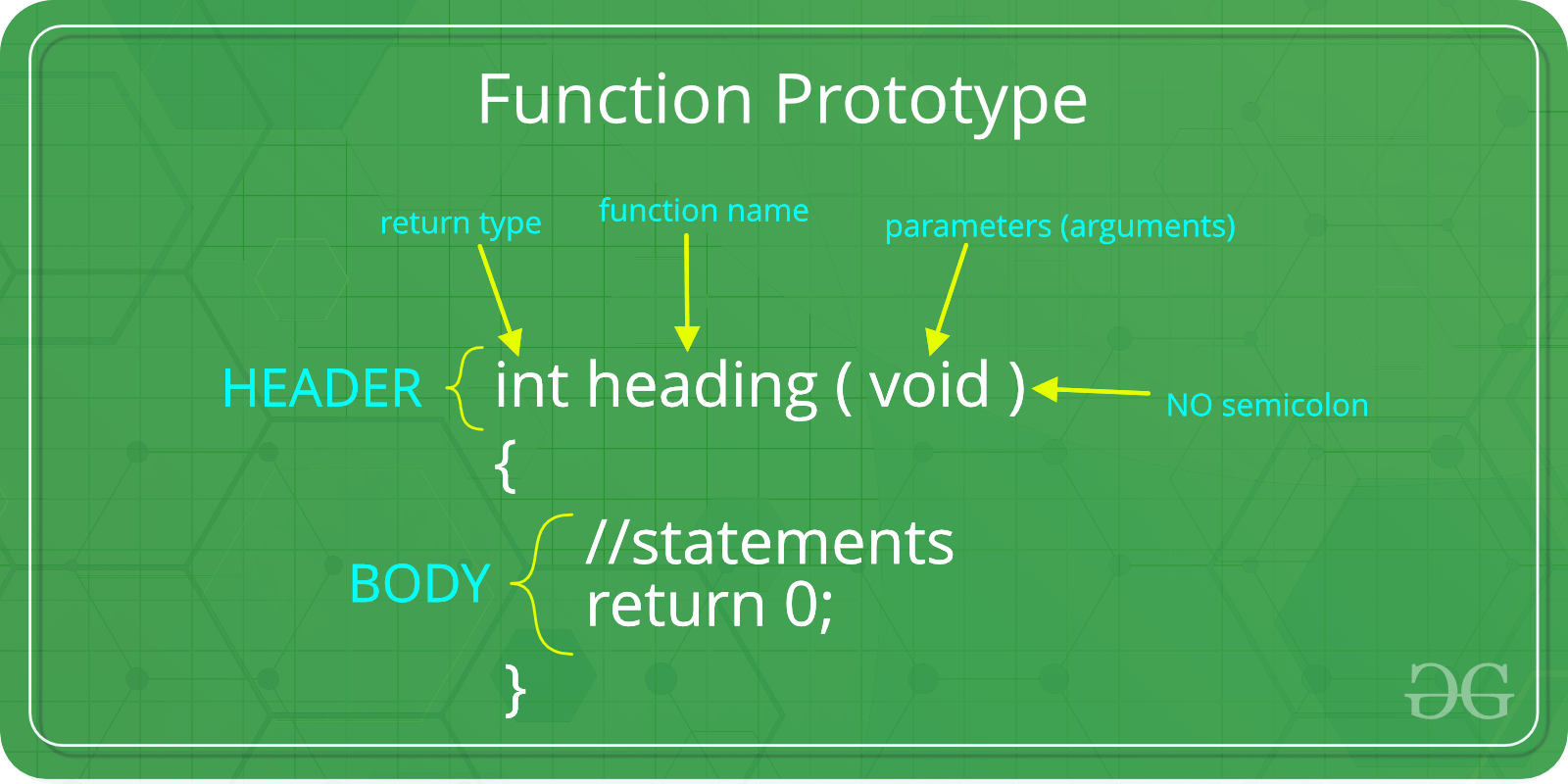# C function argument and return values

• Difficulty Level : Easy
• Last Updated : 30 May, 2022

Prerequisite: Functions in C/C++ A function in C can be called either with arguments or without arguments. These functions may or may not return values to the calling functions. All C functions can be called either with arguments or without arguments in a C program. Also, they may or may not return any values. Hence the function prototype of a function in C is as below: |1. Function with no argument and no return value: When a function has no arguments, it does not receive any data from the calling function. Similarly, when it does not return a value, the calling function does not receive any data from the called function. Syntax :
```Function declaration : void function();
Function call : function();
Function definition :
void function()
{
statements;
}```

## C

 `// C code for function with no` `// arguments and no return value`   `#include ` `void` `value(``void``);` `void` `main()` `{` `    ``value();` `}` `void` `value(``void``)` `{` `    ``float` `year = 1, period = 5, amount = 5000, inrate = 0.12;` `    ``float` `sum;` `    ``sum = amount;` `    ``while` `(year <= period) {` `        ``sum = sum * (1 + inrate);` `        ``year = year + 1;` `    ``}` `    ``printf``(``" The total amount is %f:"``, sum);` `}`

Output

` The total amount is 8811.708984:`
1. Function with arguments but no return value: When a function has arguments, it receives any data from the calling function but it returns no values. Syntax :
```Function declaration : void function ( int );
Function call : function( x );
Function definition:
void function( int x )
{
statements;
}```

## C

 `// C code for function` `// with argument but no return value` `#include `   `void` `function(``int``, ``int``[], ``char``[]);` `int` `main()` `{` `    ``int` `a = 20;` `    ``int` `ar = { 10, 20, 30, 40, 50 };` `    ``char` `str = ``"geeksforgeeks"``;` `    ``function(a, &ar, &str);` `    ``return` `0;` `}`   `void` `function(``int` `a, ``int``* ar, ``char``* str)` `{` `    ``int` `i;` `    ``printf``(``"value of a is %d\n\n"``, a);` `    ``for` `(i = 0; i < 5; i++) {` `        ``printf``(``"value of ar[%d] is %d\n"``, i, ar[i]);` `    ``}` `    ``printf``(``"\nvalue of str is %s\n"``, str);` `}`

Output

```value of a is 20

value of ar is 10
value of ar is 20
value of ar is 30
value of ar is 40
value of ar is 50

value of str is geeksforgeeks
```
1. Function with no arguments but returns a value: There could be occasions where we may need to design functions that may not take any arguments but returns a value to the calling function. An example of this is getchar function it has no parameters but it returns an integer and integer type data that represents a character. Syntax :
```Function declaration : int function();
Function call : function();
Function definition :
int function()
{
statements;
return x;
}
```

## C

 `// C code for function with no arguments` `// but have return value` `#include ` `#include `   `int` `sum();` `int` `main()` `{` `    ``int` `num;` `    ``num = sum();` `    ``printf``(``"\nSum of two given values = %d"``, num);` `    ``return` `0;` `}`   `int` `sum()` `{` `    ``int` `a = 50, b = 80, sum;` `    ``sum = ``sqrt``(a) + ``sqrt``(b);` `    ``return` `sum;` `}`

Output

`Sum of two given values = 16`
1. Function with arguments and return value Syntax :
```Function declaration : int function ( int );
Function call : function( x );
Function definition:
int function( int x )
{
statements;
return x;
}```

## C

 `// C code for function with arguments` `// and with return value`   `#include ` `#include ` `int` `function(``int``, ``int``[]);`   `int` `main()` `{` `    ``int` `i, a = 20;` `    ``int` `arr = { 10, 20, 30, 40, 50 };` `    ``a = function(a, &arr);` `    ``printf``(``"value of a is %d\n"``, a);` `    ``for` `(i = 0; i < 5; i++) {` `        ``printf``(``"value of arr[%d] is %d\n"``, i, arr[i]);` `    ``}` `    ``return` `0;` `}`   `int` `function(``int` `a, ``int``* arr)` `{` `    ``int` `i;` `    ``a = a + 20;` `    ``arr = arr + 50;` `    ``arr = arr + 50;` `    ``arr = arr + 50;` `    ``arr = arr + 50;` `    ``arr = arr + 50;` `    ``return` `a;` `}`

Output

```value of a is 40
value of arr is 60
value of arr is 70
value of arr is 80
value of arr is 90
value of arr is 100
```

My Personal Notes arrow_drop_up
Recommended Articles
Page :